Next: Inversion of the Hessian Up: Least-squares solution of the Previous: Least-squares solution of the

# Inversion of a 22 block matrix

Let us define theblock matrix M as follows: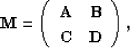(87)
where A, B, C, and D are matrices. First, we consider the matrix equation(88)
If we multiply the top row by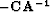and add it to the bottom, we have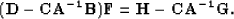(89)
Then we can easily find F and E. The quantity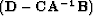is called the Schur complement of A and, denoted as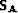, appears often in linear algebra Demmel (1997). The derivation of F and E can be written in a matrix form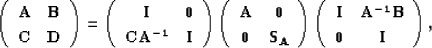(90)
which resembles an LDU decomposition of M. Alternatively, we have the UDL decomposition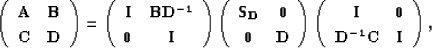(91)
whereis the Schur complement of D. The inversion formulas are then easy to derive as follows:(92)
and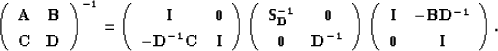(93)
The decomposition of the matrix M offers opportunities for fast inversion algorithms. The final expressions for M are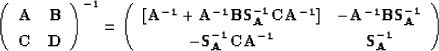(94)
and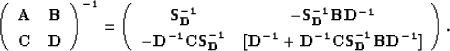(95)
Equations () and () yield the matrix inversion lemma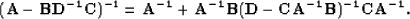(96)Next: Inversion of the Hessian Up: Least-squares solution of the Previous: Least-squares solution of the
Stanford Exploration Project
5/5/2005